Journal of Integer Sequences, Vol. 7 (2004), Article 04.1.6

## Partial Complements and Transposable Dispersions

### Clark Kimberling and John E. Brown Department of Mathematics University of Evansville 1800 Lincoln Avenue Evansville, IN 47722 USA

Abstract:

Suppose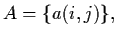for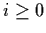and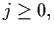is the dispersion of a strictly increasing sequence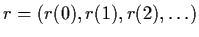of integers, where r(0)=1 and infinitely many postive integers are not terms of r. Let R be the set of such sequences, and define t on R by tr(n)=a(0,n) for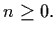Let F be the subset of R consisting of sequences r satisfying ttr=r. The set F is characterized in terms of ordered arrangements of numbers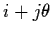. For fixed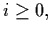the sequence a(i,j), for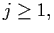is the (i+1)st partial complement of r. Central to the characterization of F is the role of the families of figurate (or polygonal) number sequences and the centered polygonal number sequences. Finally, it is conjectured that for every r in R, the iterates t(2m)r converge to a sequence in F.

Full version:  pdf,    dvi,    ps,    latex

(Concerned with sequences A000124 A000217 A000290 A000326 A001401 A001840 A001844 A001972 A002061 A002620 A005448 A008748 A077043 A082152 A082153 A082154 A082155 A082156 A086270 A086271 A086272 A086273 A087465 A087466 A087467 A087468 A087469 A087470 .)

Received October 14 2003; revised version received January 19 2004. Published in Journal of Integer Sequences February 19 2004.